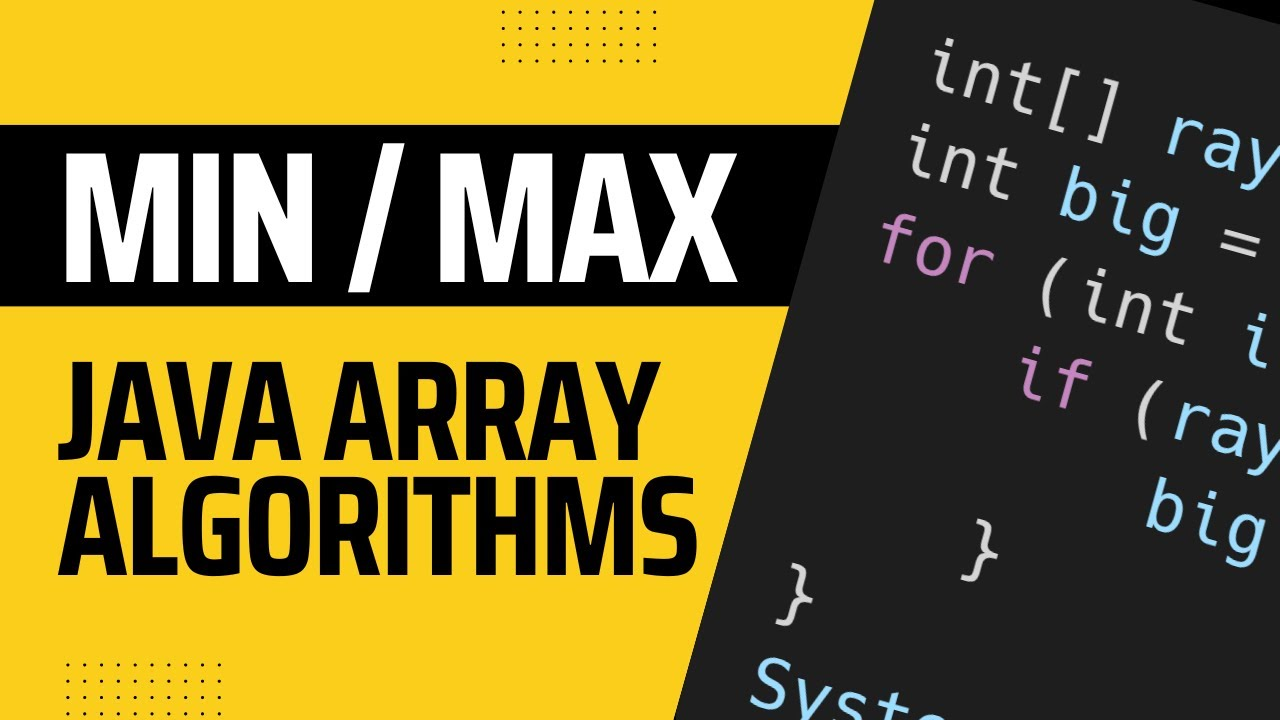# Minimum / Maximum algorithm in Java

Let’s say you’re taking a class and the teacher drops your lowest daily grade. Odds are really good that they’re not manually going through everybody’s grades and finding the lowest value. They’re probably letting the gradebook software take care of it, and that software is using some type of algorithm to find the lowest value.

## Smallest Value Algorithm

``````public int minValue(int[] ray) {
int min = Integer.MAX_VALUE;
for (int i = 0; i < ray.length; i++) {
if (ray[i] < min) {
min = ray[i];
}
}
return min;
}
``````

Let’s look at what’s going on here.

First, we create a variable `min` that will hold the smallest value that we’ve currently found. It starts at `Integer.MAX_VALUE` which is the largest possible value that an `int` can hold. That means every value in `ray` cannot be larger.

Next we loop through the array and compare every value to the current smallest value. If we find that `ray[i]` is smaller than `min`, we set `min` to be equal to `ray[i]` as it’s our new lowest value.

Finally, we return the smallest value that we found.

### Another Way

If you know that there is at least one value in `ray`, and it would be sort of silly for there not to be, you can set `min` to be equal to `ray` and then start your loop at `i = 1`. That way you don’t have to check the first value against `Integer.MAX_VALUE`.

It’d look almost the same.

``````public int minValue(int[] ray) {
int min = ray;
for (int i = 1; i < ray.length; i++) {
if (ray[i] < min) {
min = ray[i];
}
}
return min;
}
``````

And if you’re a for each loop enjoyer, you can do it that way as well.

``````public int minValue(int[] ray) {
int min = Integer.MAX_VALUE;
for (int i : ray) {
if (i < min) {
min = i;
}
}
return min;
}
``````

This time we do start at the first element because that’s the way for each loops work.

## Largest Value Algorithm

Finding the largest value is almost exactly the same as finding the smallest value. We do want to start with a really small value this time, so we’ll use `Integer.MIN_VALUE` instead of `Integer.MAX_VALUE`. And the comparison flips from `<` to `>`.

``````public int maxValue(int[] ray) {
int max = Integer.MIN_VALUE;
for (int i = 0; i < ray.length; i++) {
if (ray[i] > max) {
max = ray[i];
}
}
return max;
}
``````

And the same two extra examples above starting with `max = ray` and then starting the loop at index `1` or using a for each loop would work here as well.

## VideoThis site contains affiliate links. If you click an affiliate link and make a purchase we may get a small commission. It doesn't affect the price you pay, but it is something we must disclose.